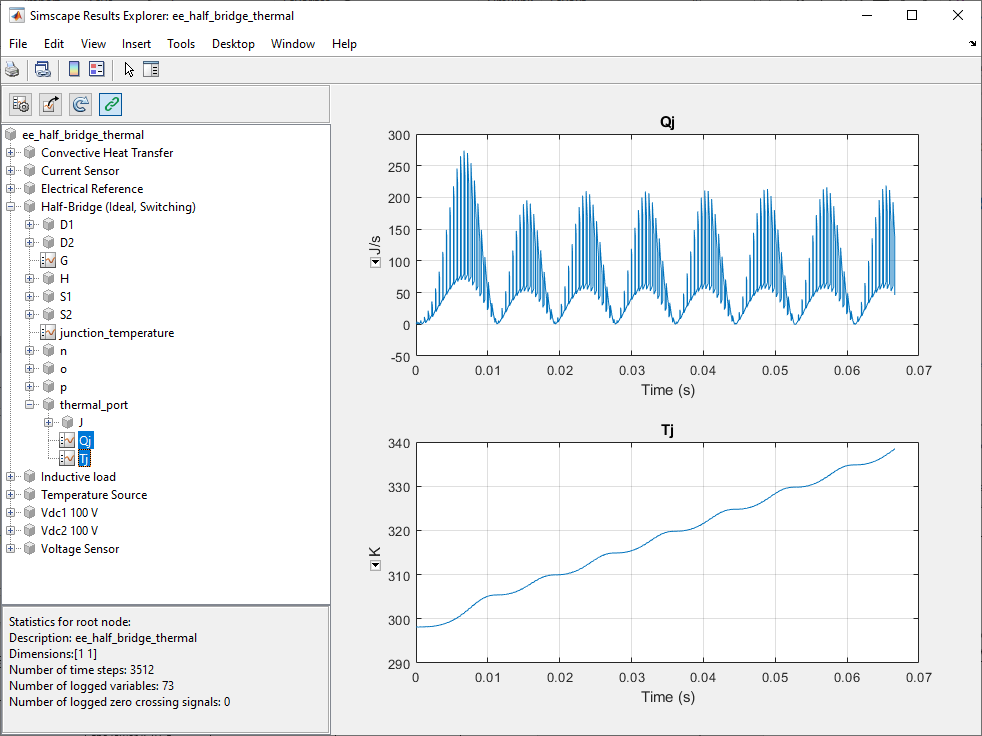## Build and Simulate Single-Phase Half-Bridge Inverter With Ideal Switches and Thermal Port

In this example, you build and analyze a single-phase, half-bridge inverter that you control by using sinusoidal pulse-width modulation (SPWM) and simulate the effects of generated heat and device temperature.

To see the completed version of the model you create in this example, at the MATLAB command prompt, enter `ee_half_bridge_thermal`.

### Build the Model

The single-phase, half-bridge inverter in this example consists of a power circuit and a control system. First, create both parts of the model by adding and connecting the blocks.

#### Build the Power Circuit

The power circuit comprises a DC link, two semiconductor switches with their anti-parallel diode, and an inductive load.

To build the power circuit:

1. Create a new model.

2. Add the blocks in this table. The Library Path column specifies the hierarchical path to each block.

Block

Purpose

Library Path

Quantity

DC Voltage SourceProvide a DC linkSimscape > Foundation Library > Electrical > Electrical Sources

2

Half-Bridge (Ideal, Switching)Model two semiconductor switches with their anti-parallel diodes and build the half-bridge with thermal effects

Simscape > Electrical > Semiconductor & Converters

1

InductorModel an inductive loadSimscape > Foundation Library > Electrical > Electrical Elements

1

Convective Heat TransferRepresent a heat transfer by convection from the half-bridge to the ambientSimscape > Foundation Library > Thermal > Thermal Elements

1

Temperature SourceDefine the ambient temperatureSimscape > Foundation Library > Thermal > Thermal Sources

1

Electrical ReferenceProvide the ground connection for the electrical conserving portsSimscape > Foundation Library > Electrical > Electrical Elements

1

Voltage SensorMeasure the voltage across the loadSimscape > Foundation Library > Electrical > Electrical Sensors

1

Current SensorMeasure the current that flows through the loadSimscape > Foundation Library > Electrical > Electrical Sensors

1

Solver ConfigurationDefine the solver settings that apply to all physical modeling blocksSimscape > Utilities

1

Simulink-PS ConverterConvert an output physical signal to a Simulink signalSimscape > Utilities

2

PS-Simulink Converter Convert a Simulink input signal into physical signalSimscape > Utilities

2

ScopeDisplay the load voltage and the current waveSimulink > Commonly Used Blocks

2

Note

You can use the Simscape™ function `ssc_new` with a domain type of `electrical` to create a Simscape model that contains these blocks:

• Scope

• Solver Configuration

• Electrical Reference

3. Rename and connect the blocks as shown in the diagram.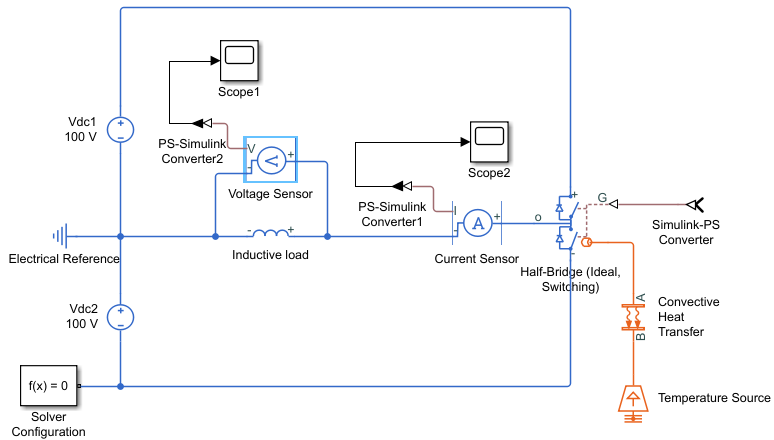To build the power circuit, this example uses a Half-Bridge (Ideal, Switching) block instead of an IGBT (Ideal, Switching) block. Similarly to the Half-Bridge (Ideal, Switching) block, you can calculate and parameterize the conduction loss of the IGBT and its anti-parallel diode, and the switching-on and switching-off losses of the IGBT. However, you cannot calculate the reverse recovery loss of the diode by using the same lookup table approach. To account for the reverse recovery loss, you need to specify the parameters associated with the diode charging dynamics. If you include the diode charging dynamics, the simulation requires more computational effort, compromising its performance.

#### Build the Control

The control system generates two pulse-width modulated (PWM) gate signals that control the switching behavior of two IGBTs in the Half-Bridge (Ideal, Switching) block.

To build the control system:

1. Add the blocks in this table to the model. The Library Path column specifies the hierarchical path to each block.

Block

Purpose

Library Path

Quantity

Sine WaveGenerate a sinusoidal signal that represents a unit sinusoidal modulation waveSimulink > Sources1
ConstantDefine the sinusoidal pulse-width modulation indexSimulink > Sources1
ProductObtain the sinusoidal modulation wave by multiplying the unit sinusoidal wave by the modulation indexSimulink > Math Operations

1

BiasPerform calculation to obtain the duty cycle signalSimulink > Math Operations

1

1

PWM GeneratorGenerate PWM gate signal for the upper IGBT of the Half-Bridge (Ideal, Switching) blockSimscape > Electrical > Control > Pulse Width Modulation

1

Logical OperatorObtain the complementary PWM gate signal for the lower IGBT of the Half-Bridge (Ideal, Switching) blockSimulink > Logic and Bit Operations

1

Data Type ConversionConvert the input Boolean signal to double signalSimulink > Signal Attributes

1

Goto Pass the input PWM gate signal to the corresponding From blockSimulink > Signal Routing

2

FromReceive a PWM gate signal from the corresponding Goto block and pass the signal as an outputSimulink > Signal Routing

2

MuxCombine two PWM gate signalsSimulink > Signal Routing

1

2. Rename and connect the blocks as shown in the diagram.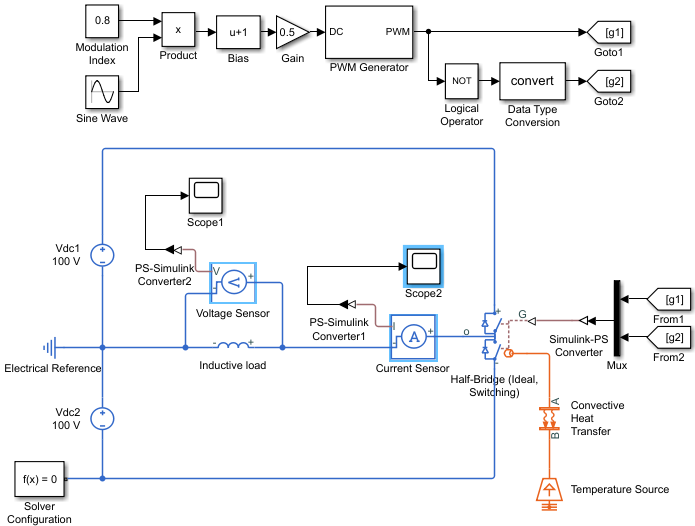### Specify Model Parameters

Specify these parameters to represent the behavior of the system components:

#### Model Setup Parameters

These blocks specify model information that is not specific to a particular block:

• Solver Configuration

• Electrical Reference

As with Simscape models, you must include a Solver Configuration block in each topologically distinct physical network. This example has a single physical network, so use one Solver Configuration block with the default parameter values.

You must include an Electrical Reference block in each Simscape Electrical™ network. This block does not have any parameters.

#### Power Circuit Parameters

Specify these parameters to represent the behavior of the power circuit components:

1. Set the Constant voltage parameter of the two DC Voltage Source blocks to `100`.

2. In the Half-Bridge (Ideal, Switching) block, set the parameters as follows:

• Main settings

• Gate-control port`PS`

• Switching device`IGBT`

• Threshold voltage, Vth`0.5`. With this value, the Half-Bridge (Ideal, Switching) switches on when the value at the input port is equal to `1` and switches off when the value at the input port is equal to `0`. If you set the threshold voltage to the actual threshold value, then you must change the value at the input gate ports accordingly.

• On-state behavior and losses```Tabulate with temperature and current```

• Integral Diode settings

• Integral protection diode`Yes`

• Diode model```Tabulated I-V curve```

• Table type```Table in If(Tj,Vf) form```

• Thermal Port settings

• Thermal network`External`

• Junction thermal mass`0.1`

• Variables settings

• Select Override Junction temperature Target.

• Priority`High`

• Value`25`

3. In the Inductor block, set these parameters:

• Inductance`0.005`

• Series resistance`1`

• Parallel conductance`0`

4. In the Convective Heat Transfer block, set these parameters:

• Area`1e-3`

• Heat transfer coefficient`20`

5. In the Temperature Source block, set the Temperature parameter to `298.15`.

For a semiconductor device, the generated heat, or thermal loss, includes:

• Conduction loss of the switch and its anti-parallel diode

• Switching-on and switching-off loss of the switch

• Reverse recovery loss of the anti-parallel diode

In this model, the Half-Bridge (Ideal, Switching) block models the semiconductor. The example models the thermal loses based on the voltage, current, and temperature. When the Half-Bridge (Ideal, Switching) block conducts current, the conduction loss is calculated by multiplying the on-state voltage across the half-bridge and the current that flows through itself.

A 2-D lookup table determines the value of the on-state voltage. To define the 2-D lookup table, in the Main settings, specify the values of the On-state voltage Vce(Tj,Ice), Temperature vector Tj, and Collector-emitter current vector Ic parameters.

The conduction loss of the anti-parallel diode is calculated by using the Forward currents If(Tj,Vf), Junction temperatures, Tj, and Forward voltages, Vf parameters in the Integral Diode settings.

A 2-D lookup table determines the value of the switching-on loss of the IGBT and then proportionates it to the Off-state voltage for loss data parameter. To define the 2-D lookup table, in the Losses settings, specify the values of the Switch-on loss, Eon(Tj,Ice), Temperature vector for losse,s Tj, Collector-emitter current vector for losses, Ice parameters.

The switching-off loss of the IGBT is calculated by using the Switch-off loss, Eoff(Tj,Ice) parameter in the Losses settings and the reverse recovery loss of the diode is calculated by using the Diode reverse recovery loss, Erec(Tj,Ice) parameter.

The generated heat dissipates from the half-bridge to the ambient atmosphere. The Convective Heat Transfer and the Temperature Source blocks model this thermal behavior. For more options of thermal network modeling, see Simulating Thermal Effects in Semiconductors.

#### Control System Parameters

Specify the following parameters to get the PWM gate signals for the upper and lower IGBTs from a sinusoidal modulation wave:

1. In the Constant block, set the Constant value parameter to `0.8`.

2. To generate a unit sinusoidal modulation wave, set the parameters of the Sine Wave block:

• Amplitude`1`

• Bias`0`

• Frequency (rad/sec)`2*pi*60`

• Phase (rad)`0`

• Sample time`0`

3. In the Bias block, set the Bias parameter to `1`.

4. In the Gain block, set the Gain parameter to `0.5`.

5. Set the PWM Generator block parameters:

• Carrier counter`Up-Down`

• Timer period (s)`1/2/2000`

• Phase delay (s)`0`

• Sample time`0`

6. In the Logical Operator block, set the Operator parameter to `NOT`.

The sinusoidal modulation wave is equal to $0.8\cdot \mathrm{sin}\left(2\pi \cdot 60\cdot t\right).$ To change the modulation wave, modify the modulation index, frequency, and phase values.

The PWM Generator block cannot receive the modulation wave as an input, because it must be a duty cycle. To obtain the duty cycle from the modulation wave, this example uses this equation:

`$D=\frac{M+1}{2}$`

where D is the duty cycle and M is the modulation wave. The Bias and Gain blocks of the control system implement this calculation.

The output PWM gate signal from the PWM Generator block controls the upper IGBT. The Logical Operator block implements the complementary PWM gate signal that controls the lower IGBT. The value of the Timer period (s) parameter of the PWM Generator block is equal to `1/2/2000`, which means that the switching frequency is equal to `2` `kHz`.

The Goto blocks and From blocks implement the connection between the generated PWM gate signals and the two IGBTs in the Half-Bridge (Ideal, Switching) block. Setting the Goto tag parameter in a Goto and From block to the same tag allows you to pass a signal from one block to another without connecting them.

#### Signal Display Parameters

Specify the parameters of the Scope block to display the output signals.

Double-click the Scope blocks and then click the View > Configuration Properties to open the Scope Configuration Properties dialog box. On the Logging tab, clear the Limit data points to last check box.

### Configure the Solver Parameters

Configure the solver parameters to use a continuous-time solver. Simscape Electrical models only run with a continuous-time solver when you clear the Local Solver parameter of a Solver Configuration block. Use the Configuration Parameters dialog to change the simulation end time, tighten the relative tolerance for a more accurate simulation, and remove the limit on the number of simulation data points Simulink® saves.

1. In the model window, select Modeling > Model Settings to open the Configuration Parameters dialog box.

2. In the left pane, click Solver:

• Set Stop time to `4/60`.

• Set Solver to ```ode23t (Mod. stiff/Trapezoidal)```.

• Set Max step size to `1e-4`.

• Set Relative tolerance to `1e-4`.

3. Click OK.

### Simulate the Model and Analyze the Results

Run the simulation and plot the results. In the model window, select SimulationRun.

To view the load voltage and current, double-click the Scope blocks. You can do this before or after you run the simulation.

This figure shows the load current.The next figure shows the load voltage.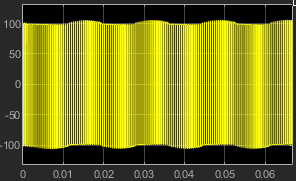The load voltage is a PWM wave varying between -100 V and 100 V.

To view other simulation results, such as the conduction loss of the Half-Bridge (Ideal, Switching) block, use the Simscape Results Explorer. To enable the Simscape Results Explorer, first log the simulation data for all variables:

• In the model window, select Modeling > Model Settings to open the Configuration Parameters dialog box.

• In the left pane, click Simscape:

• Set Log simulation data to `All`.

• Select the Open viewer after simulation check box.

• Clear the Limit data points check box.

• Click OK.

In the model window, select Simulation > Run to run the simulation. This figure shows the Simscape Results Explorer window that opens automatically.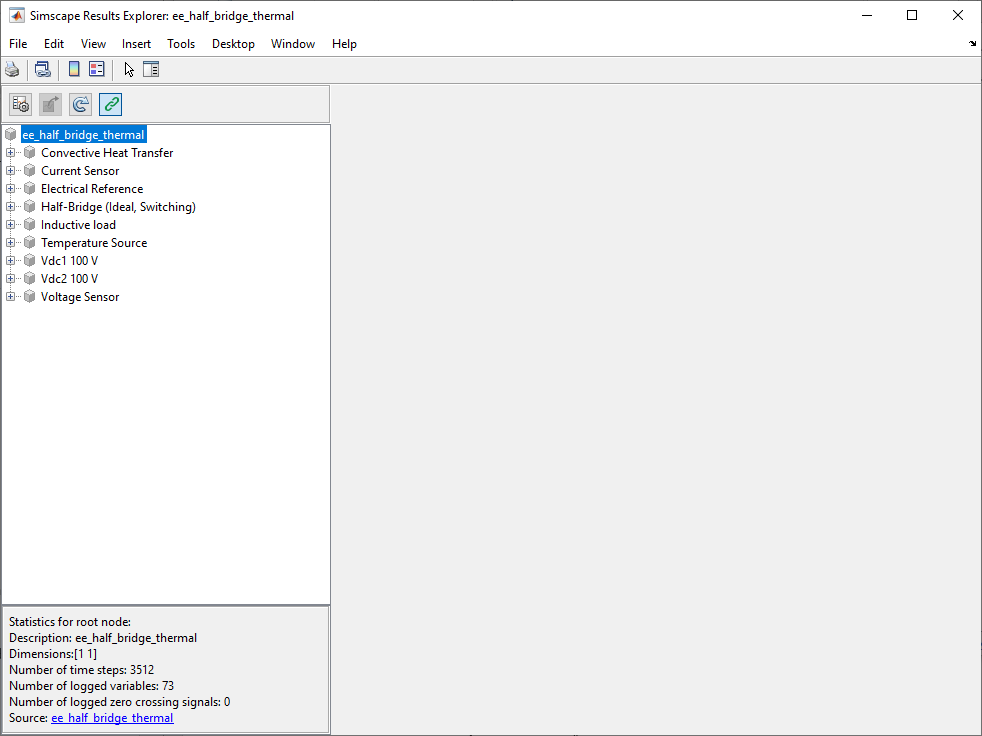When you click a node in the left pane, the corresponding plots appear in the right pane. For example, if you click the variable power_dissipated in Half-Bridge (Ideal, Switching) > S1, then the Simscape Results Explorer plots the conduction loss of the upper IGBT in the half-bridge.To select several variables for side-by-side plot comparison, press Ctrl and click multiple variables. For example, check the switching-on and switching-off loss of the upper IGBT and the reverse recovery loss of the anti-parallel diode: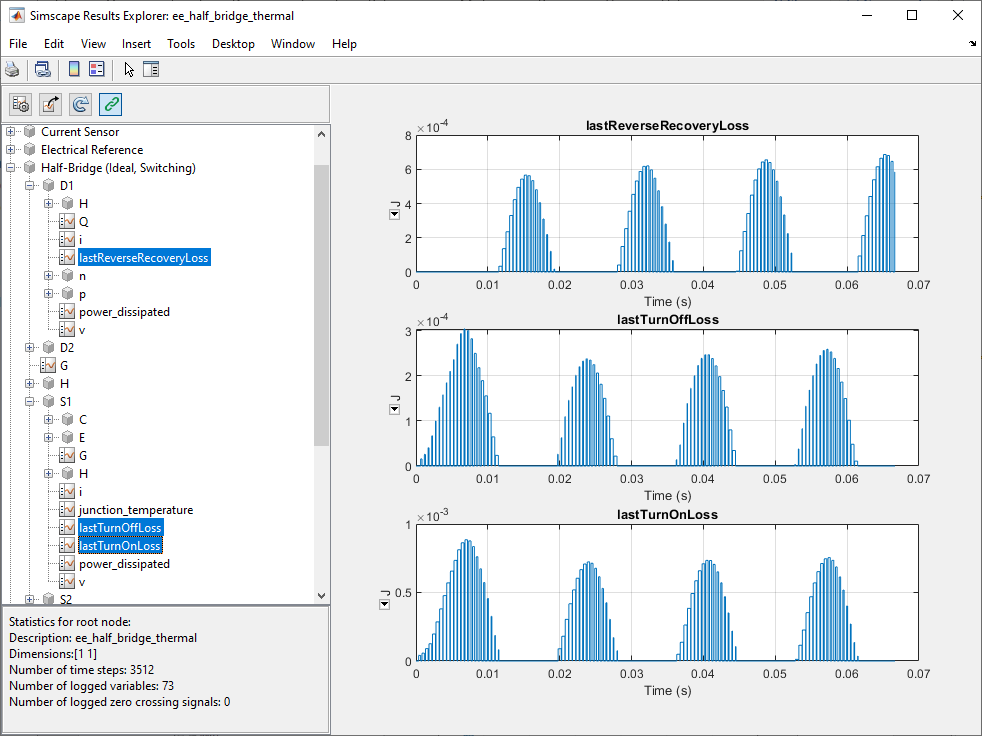You can also check the total power loss and the junction temperature of the half-bridge by clicking the Qj and Tj variables in Half-Bridge (Ideal, Switching) > thermal_port.